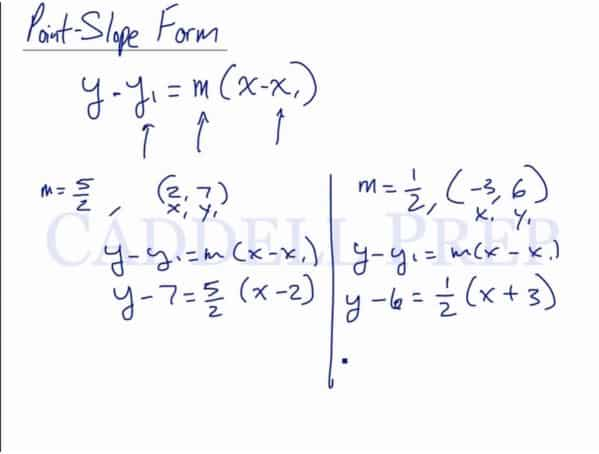In the video above, you will learn about the point-slope form of a line and why it’s useful. After you finish this lesson, view all of our Algebra 1 lessons and practice problems.

What is the point-slope form of a line?

The point-slope form is written in the format:$y - y_1 = m(x - x_1)$

If you are given a point that a line passes though,$(x_1, y_1)$, and the slope$m$, you can substitute the values into the formula. Do not substitute any values in for$x$ and$y$.

Point-slope form is useful when you know the slope of a line and a point it passes through. Substituting the slope and coordinates in, you are able to derive other forms of the equation of a line, such as slope-intercept.

Here’s an example:$m = \frac{1}{2}$$x_1 = 6$$y_1 = -3$

In point slope format, this would be written as:$y - (6) = \frac{1}{2}(x - (-3))$$y - 6 = \frac{1}{2}(x+3)$

Find Point Slope Form with Two Points

It’s possible to find point slope form without being given the slope. However, you will need two points that the line passes through instead of just one.

The formula only requires one point,$(x_1, y_1)$, but we need two points so we can find the slope.

Use the slope formula to find the slope.$m= \dfrac{y_2-y_1}{x_2-x_1}$

After finding the slope, substitute it into the point slope form formula along with one of the points. You can use either point; it doesn’t matter which one you use.

Example of Point-Slope Form Of A Line

Example 1$m = 5$$x_1 = 3$$y_1 = 2$

In point slope format, this would be written as:$y - (2) = 5(x - 3)$$y - 2 = 5x+15$

Example 2$m = \dfrac{2}{3}$$x_1 = 12$$y_1 = 3$

In point slope format, this would be written as:$y - (3) = \dfrac{2}{3}(x - 12)$$y - 3 = \dfrac{2}{3}x - \dfrac{2}{3}(12)$$y - 3 = \dfrac{2}{3}x - \dfrac{24}{3}$$y - 3 = \dfrac{2}{3}x - 8$

Video-Lesson Transcript

Let’s go over point slope form.

The actual equation is:$y - y_1 = m (x - x_1)$

where$m$ is the slope of the line,$x_1$ and$y_1$ are the coordinate of the line.

For example, we have:$m = \dfrac{5}{2}, (2, 7)$

Let’s solve by substituting the values$y - y_1 = m (x - x_1)$$y - 7 = \dfrac{5}{2} (x - 2)$

That’s it.

Let’s have another one.$m = \dfrac{1}{2}, (-3, 6)$$y - y_1 = m (x - x_1)$$y - 6 = \dfrac{1}{2} (x + 3)$Above are two examples of finding the point slope form of a line by substituting the slope and coordinates into the formula.

Let’s discuss this further.

We already know the formula for slope is:$m = \dfrac{y_2 - y_1}{x_2 - x_1}$

Let’s say we write this as fraction$\dfrac{m}{1} = \dfrac{y_2 - y_1}{x_2 - x_1}$

Now, let’s cross multiply. It will be$m (x_2 - x_1) = y_2 - y_1$

Then instead of writing$x_2$ and$y_2$, let’s just write them as$x$ and$y$.

Now, our equation will look like this$m (x - x_1) = y - y_1$

This is already the point-slope form, so let’s just rewrite this as$y - y_1 = m (x - x_1)$

So the point-slope form is just derived from the slope formula.

From the point-slope form, we can find the equation of a line in a slope intercept.

For example:$m = 2, (3, -1)$$y - y_1 = m (x - x_1)$$y + 1 = 2 (x -3)$

The equation of a line in a slope intercept is:$y = mx + b$

Let’s continue solving by distributing$2$ in the parenthesis.$y + 1 = 2x - 6$

Then isolate$y$$y + 1 - 1 = 2x - 6 - 1$$y = 2x - 7$

So now, our answer is the equation of a line in a slope intercept form which we derived from the point slope form.